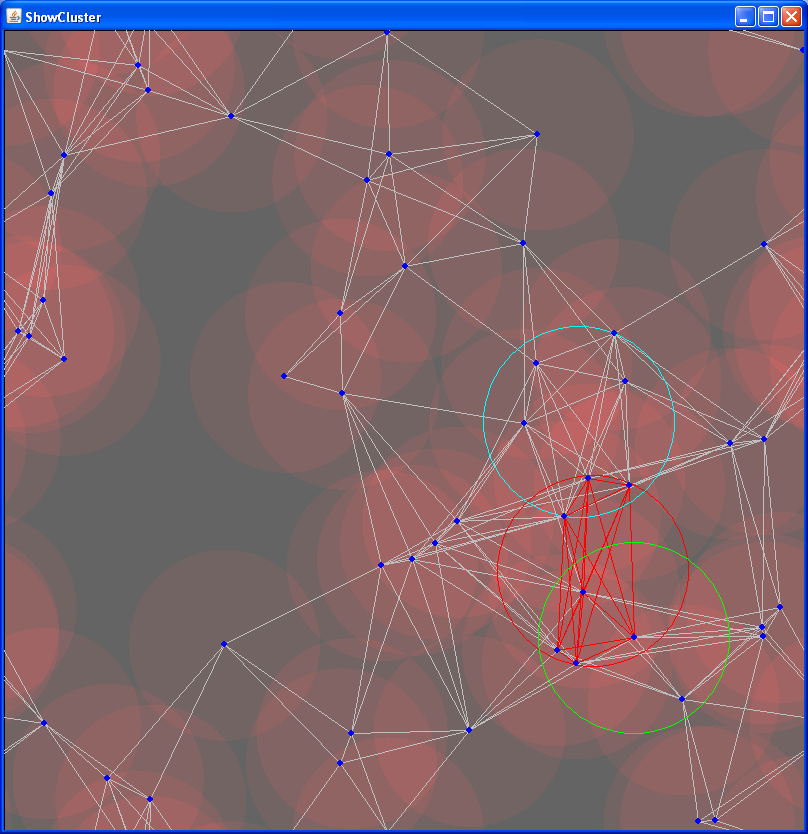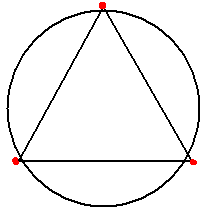# Placement of circle over points

For the Queue ICPC programming game Capture I ran into a geometrical problem.
While programming my little robot I wanted to have an algorithm calculate this for me:

1. I have a field with 122 points
2. I have a circle of fixed size

How do I calculate where to put the circle so it encapsulates the most points?

This is what I came up with, three algorithms:

# Algorithm #1: Centerpoint

The first algorithm was created as a test. It doesn’t find the perfect solution, but gives a decent solution.
First I loop over all the points, and I check if there are points located at CIRCLE_RADIUS length from our point. This returns a score. The best point has a lot of other points nearby.

This algorithm is very quick, but it has a huge disadvantage, the circle will always have one of our points as center.

It will never yield the perfect solution… In the following picture this algorithm produces the green circle:# Algorithm #2: Heatmap

The next idea I got was based on a heatmap. It is very computer heavy, but it will generate the best solution.

It works like this (psuedocode):

The pixel with the best score is used in most circles. Thus, this would need to be the center of our circle!

In the image above you can see the resulting heatmap, and the cyan circle is placed on the best possible pixel.

(In most cases, there are more then one ‘best pixels’, which one you choose doesn’t matter)

# Algorithm #3: Bron-Kerbosch-myself

The heatmap algorithm, mentioned above, takes a LOT of processing time and is far from the most efficient algorithm. For the Queue ICPC contest there is a time limit for each calculation, and I need it to speed up!

So I started to wonder:

- What do all the points in the perfect circle have in common?

Well, they all have a maximum distance to each other of CIRCLE_RADIUS * 2.

So I started to play with graph algorithms.

The lines you see in the image above are all the points that can be connected with a maximum length of CIRCLE_RADIUS * 2. All the lines show points that could possibly be in the same circle together.
Then I googled a bit and found the term ‘clique’. A clique is a group of points that all point to each other, just what we want with the graph shown in the picture!

When I searched a little further I came across the Bron-Kerbosch algorithm. It finds the perfect maximum clique for a given undirected graph! Again, just what we want!

Here is the pseudocode (from Wikipedia):

But I ran into a little problem, I’d made a false assumption… If you have a triangle of three points, with all legs size N, and then draw a circle from the center… none of the points fall into the circle.Now I tried two ways to solve this, the first one is to use a smaller distance in the graph. If you only select points that are located (cosine(30) * CIRCLE_RADIUS * 2) every point will eventually fall into our circle. But this could eliminate the perfect circle obviously if two points in the perfect solution are further apart then (cosine(30) * CIRCLE_RADIUS * 2).

Then I desected the Bron-Kerbosch algorithm, and decided to change it a bit. This is what I ended up with:

This returns all the maximal cliques that fall into our circle, and we are always sure they fall into our circle..! I think this will always generate the perfect solution, just as the heat map. In this the above picture, this algorithm produces the red circle.

It already is light years faster then the heat map algorithm, but I still need a bit more speed. I’m still struggling to improve my implementation, like trying to use a pivot as bronKerbosch2 (see wikipedia again) does.

# Algorithm #4: ???

For some reason I still fail to believe I’m the first person in the world to tackle this problem. But I haven’t been able to find an algorithm for this. Some people recommended using a sweeping algorithm but I don’t understand how this is used to find the circle’s location. Others pointed me to Voronoi maps…

So if you have any suggestions, or know the algorithm I’m looking for, please add a comment :-)